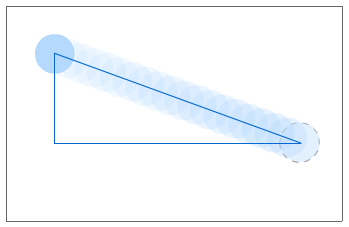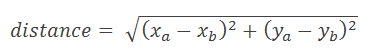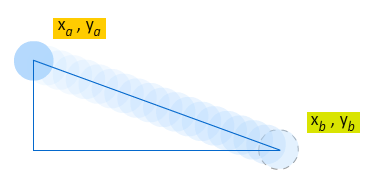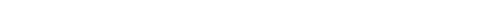# Finding the Distance Between Two Points

by kirupa  |  13 January 2011 (revised on 17 January 2011)

There will be times when you need to know how far apart two items are. While it is common in games or situations involving collision detection, you will run into needing to know the distance more often than you think. For example, the Random Movement animation is a case where the distance between two points is used to gauge when to change direction:In this short tutorial, you will learn how to use the Pythagorean Theorem to calculate the distance when you know the x and y coordinates of two points.

A full explanation and more about the Pythagorean Theorem can be found on Wikipedia, but in a nutshell, the equation looks as follows:Your distance is the square root of the net horizontal distance squared and your net vertical distance squared. Continuing the example we started off with, the xa, xb, ya, and yb values can be visualized as follows:The code for converting our equation into the ActionScript that Flash understands is as follows:

private function GetDistanceSquare(xA:Number, yA:Number, xB:Number, yB:Number):Number
{
var xDiff:Number = xA - xB;
var yDiff:Number = yA - yB;

return Math.sqrt(xDiff * xDiff + yDiff * yDiff);
}

Two simplify our expression, I create two variables called xDiff and yDiff that store the difference in the x and y positions between the two points:

var xDiff:Number = xA - xB;
var yDiff:Number = yA - yB;

Once I have the difference, the rest of the math is very straightforward:

Math.sqrt(xDiff * xDiff + yDiff * yDiff);

Below, you will find a larger example that demonstrates finding the distance between two points at (0, 100) and (0, 100):

public class MainDocument extends MovieClip
{
public function MainDocument()
{
// constructor code
var distance:Number = GetDistance(0,0,100,100);
trace(distance);
}

private function GetDistance(xA:Number, yA:Number, xB:Number, yB:Number):Number
{
var xDiff:Number = xA - xB;
var yDiff:Number = yA - yB;

return Math.sqrt(xDiff * xDiff + yDiff * yDiff);
}
}

That's all there is to finding the distance between two points. If there is anything you take away from this tutorial, just ask yourself, wasn't Pythagoras one smart dude?

Note
For calculating squares, there is the Math.pow function that could be used instead of multiplying the same values twice. The original version of this tutorial used that approach actually:

private function GetDistance(xA:Number, yA:Number, xB:Number, yB:Number):Number
{
return Math.sqrt(Math.pow(xA - xB,2) + Math.pow(yA - yB,2));
}

The reason this approach was not the one presented above is due to performance. Using the simpler multiplying approach, my informal tests showed a gain of around 30 - 40%. While it may not be accurate using the approach I used to measure this, at least it gives you a ballpark. Thanks to @_robfox who pointed this out to me via Twitter.

Got a question or just want to chat? Comment below or drop by our forums (they are actually the same thing!) where a bunch of the friendliest people you'll ever run into will be happy to help you out!